﻿ A Review of Buya’s Proof of Beal’s Conjecture and Simple Proof of Fermat's Last TheoremPublications are Open
Access in this journal
Article Versions
Export Article
• Normal Style
• MLA Style
• APA Style
• Chicago Style
Research Article
Open Access Peer-reviewed

A Review of Buya’s Proof of Beal’s Conjecture and Simple Proof of Fermat's Last Theorem

American Journal of Mathematical Analysis. 2019, 7(1), 15-16. DOI: 10.12691/ajma-7-1-3
Received October 17, 2019; Revised November 20, 2019; Accepted December 10, 2019

Abstract

In this research Buya’s proof of Beal’s conjecture will be reviewed for further improvement. It is shown that for the Beal’s conjecture problem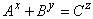in the case x = y = z = 2 A, B, and C may or may not be coprime. It is shown is shown that if each of the integers x, y, z take values greater 2, then the integers A, B and C share a common factor. In this presentation a simple proof of Fermat's last theorem is also presented using the results of proof of Beal's conjecture. Thus it is shown that Fermat's last theorem is a special case of Beal's conjecture.

1. Introduction

Beal’s conjecture was formulated in 1993 by Andrew Beal, a banker and amateur mathematician while investigating generalizations of Fermat’s last theorem. The conjecture was formulated after some extensive computational experiments were conducted in August1993. In some publications the conjecture has been occasionally referred to as the generalized Fermat’s last theorem, see 1, 2, 3, 4.

The conjecture states that:

If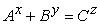where A, B, C are positive integers and x, y and z are all positive integers greater 2, then A, B and C have a common prime factor.

An algebraic relationship for proof of Beal's conjecture

Consider the Beal conjecture problem (1) below:(1)

Buya S. B. 5, 6 presented a proof of Beal's conjecture. For the purpose of this review the algebraic equation for the proof of Beal's conjecture will be given by identity equation (2) below.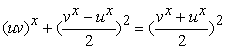(2)

In the form (2) above, and for the case x = 2, the positive integers A, B and C may or may not be coprime. The equation (2) will therefore be modified to consider non coprime cases. We will consider factoring out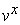so that the equation (2) takes the form (3) below.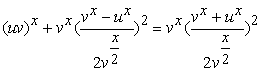(3)

In the form (3) above A, B and C have a common prime factors whenever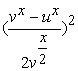and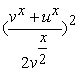are integers. Additionally, wheneverandare integers and x = 2 then at least for x = y = z = 2, A, B and C share a common prime factor.

2. Proof of Beal's Conjecture

The identity equations 2 and 3 lay the foundation for proof of Beal's conjecture. Additionally given the conditions (4) and (5) below,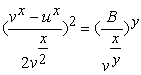(4)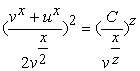(5)

The equation (2) above can be written in the form (6) below for easy proof of Beal's conjecture.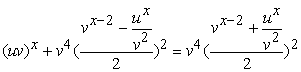(6)

In the form (6) above,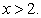If we select u and v such that they meet the conditions (7) and (8) below in which m, n, r and s are positive integers, the equation (11) takes a most general form to fully prove Beal's conjecture.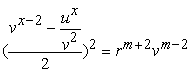(7)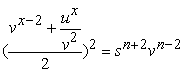(8)

The equation (6) subject to the conditions (7) and (8) above makes it possible for x, y, and z of Beal's problem to take integer values greater than 2. At the same time: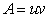(9)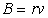(10)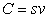(11)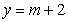(12)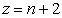(13)(14)

In this general form in which x, y and z take integer values greater than 2, the gcd of A, B and C is v. These results agree with Beal's conjecture. Thus Beal's conjecture is proved.

3. A Simple Proof of Fermat's Last Theorem

Consider the equations (4) and (5). If x = y = z, then the equations take the forms (15) and (16) below: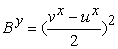(15)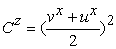(16)

The implications of equations (6) and (7) on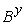and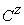are as expressed in equations (17) and (18) below:(17)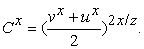(18)

On substituting (17) and (18) into equation (3) we obtain the equation (19) below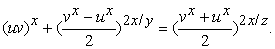(19)

From the powers of equation if we set (19)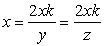and substitute back to the same equation then we obtain the equation (20) below.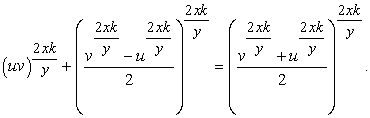(20)

For equation (20) to remain an identity then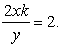This proves Fermat’s last theorem. The claim by Fermat of existence of a simple proof of his last theorem is verified.

4. Conclusion

Beal's conjecture is proved. There exists an algebraic identity that can be used to prove Beal's conjecture. The results of the proof of Beal's conjecture can be used to come up with a simple proof of Fermat's last theorem. Beal's conjecture is thus a generalization of Fermat's last theorem.

Beal's conjecture is qualified as a true theorem and qualifies to be called Beal's theorem. Fermat's claim of existence of a simple proof of his last theorem is verified.

Conflict of Interests

The author declares that there is no conflict of interest.

References

  Bennet MA, Chen I, Dahmen SR, Yazdani S (2014). Generalized Fermat Equations: A Miscellany. Simon Fraser University. In article View Article  Elkies ND (2007). The ABC's of Number theory. The Havard College mathematics Review 1(1). In article  Mauldin RD (1997). A Generalization of Fermat's last theorem: Beal’s conjecture and the Prize Problem. Notices of The AMS 44(11):1436-1437. In article  Waldschmidt M (2004). Open Diophantine problems. Moscow mathematics 4:245-305. In article View Article  Buya SB (2017a). Simple Algebraic proofs of Fermat's last theorem. Advances in Applied Science Research 8(3): 60: 64. In article  Buya SB (2017b). Solution of the Congruent number problem and proof of Birch Swinnerton-Dyer conjecture. Academia.edu. In articleThis work is licensed under a Creative Commons Attribution 4.0 International License. To view a copy of this license, visit http://creativecommons.org/licenses/by/4.0/